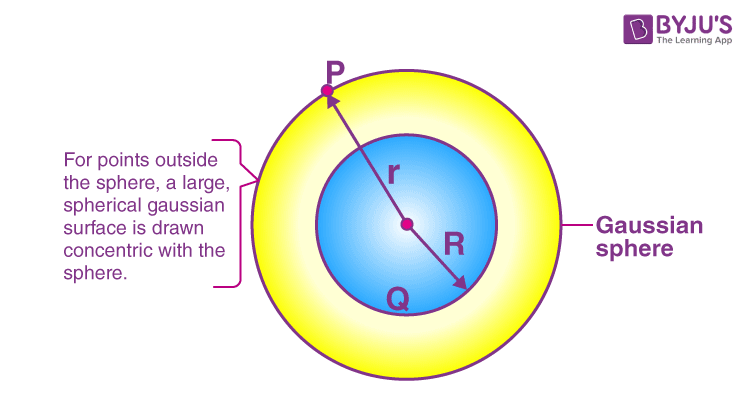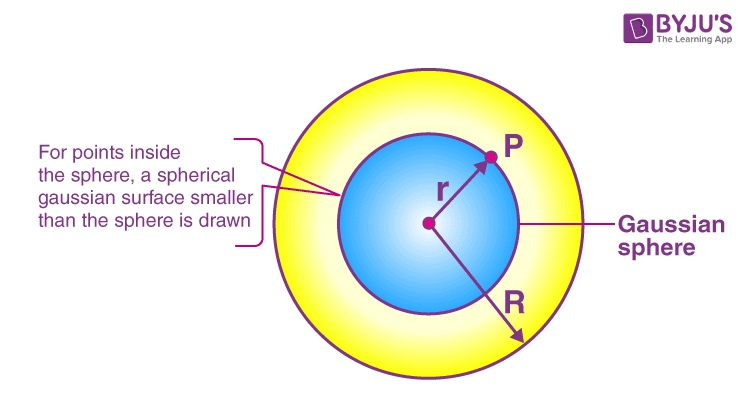Checkout JEE MAINS 2022 Question Paper Analysis : Checkout JEE MAINS 2022 Question Paper Analysis :

To determine the electric field due to a uniformly charged thin spherical shell, the following three cases are considered:

Case 1: At a point outside the spherical shell where r > R.

Case 2: At a point on the surface of a spherical shell where r = R.

Case 3: At a point inside the spherical shell where r < R.

Let us consider each case separately to determine the electric field.

Case 1: At a point outside the spherical shell where r > R.

Let P be the point outside the shell at a distance r from the centre. Since the surface of the sphere is spherically symmetric, the charge is distributed uniformly throughout the surface.A spherical Gaussian surface with the radius r and total charge enclosed on this Gaussian surface Q is selected. If Q > 0, then the electric field is radially pointed outward and if Q < 0, then the electric field is radially pointed inward.

From Gauss law, we know that

$$\begin{array}{l}\oint \vec{E}.d\vec{A}=\frac{Q}{\epsilon _{0}}\end{array}$$
(eq.1)

We can say that,

$$\begin{array}{l}E\oint_{Gaussian\;surface} dA=\frac{Q}{\epsilon _{0}}\end{array}$$
(eq.2)

This is because the electric field

$$\begin{array}{l}\vec{E}\end{array}$$
and the point
$$\begin{array}{l}d\vec{A}\end{array}$$
are in the same direction at all points on the Gaussian surface. Also, the magnitude at all the points is the same as the charge distribution is spherically symmetric.

However,

$$\begin{array}{l}\oint _{Gaussian\;surface}dA=total\;area\;of\;Gaussian\;surface=4\pi r^{2}\end{array}$$

Substituting the above in eq.2, we get

E.4πr2 = Q/ε0

E = 1/4πε0 Q/r2

In the vector form

$$\begin{array}{l}\vec{E}=\frac{1}{4\pi \epsilon _0}\frac{Q}{r^{2}}\hat{r}\end{array}$$
(eq.3)

From eq.3 we can say that the electric field at a point outside the shell will remain the same if the entire charge Q is concentrated at the centre of the spherical shell.

Case 2: At a point on the surface of a spherical shell where r = R.

When r = R, then the electric field points on the spherical shell are given as

$$\begin{array}{l}\vec{E}=\frac{Q}{4\pi \epsilon _0R^{2}}\hat{r}\end{array}$$
(eq.4)

Case 3: At a point inside the spherical shell where r < R.Let point P at a r distance from the centre inside the shell.

From Gauss law,

$$\begin{array}{l}\oint_{Gaussian\;surface}\vec{E}.d\vec{A}=\frac{Q}{\epsilon _{0}}\end{array}$$

E.4πr2 = Q/ε0 (eq.5)

We know that the Gaussian surface does not enclose any charge, therefore, Q = 0.

Therefore, the eq.5 is rewritten as

E = 0

Hence, we can say that the electric field due to the uniformly charged thin spherical shell is zero at all the points inside the shell.

## Frequently Asked Questions – FAQs

### When do we use a pillbox?

A pillbox is used when the symmetry of the charge distribution is translational along the plane.

### What are the three types of symmetry to be considered to determine the electric field?

The following are the three types of symmetry to be considered to determine the electric field:

• Planar symmetry
• Cylindrical symmetry
• Spherical symmetry

### Is Gauss law valid for any closed and open surfaces?

The Gauss law is applicable for any closed surfaces and not for open surfaces.

### What will be the charge inside the surface, if the flux of the electric field through a closed surface is zero?

The charge inside the surface will be zero if the flux of the electric field through a closed surface is zero.

### What is the SI unit of electric flux?

The SI unit of electric flux is volt meters.

## Detailed explanation of Electrostatics